# Magic Potter

A magic game based on Raspberry Pi
A Project By Huan Yang(hy468) and Hao Chen(hc795)

## Introduction

Magic Potter is a player VS player game based on Raspberry Pi, each player will have a magic wand and wave it in front of the camera. The system will recognize the trajectory of the wand and tansform into the corresponding spell. There were a total of three rounds in which each player waved his wand in front of the camera in turn. To show the game more clearly, the battle process and the winner will display on the Pi TFT. And for the relationship between different spells, it needs players to explore. For each round, the stronger spell wins.

## Project Objective

• Achieve the recognition of the wand trajectory effciently
• Show the game process on pi TFT in details
• Corresponding led will shine when recognize the spells

• ## Design

At first, we planned to make a water gun, when the system recognize the corresponding trajectory, it will shoot out water to the user. However, after consideration, we afraid that the water may lead the system to short circuit, which is dangerous. And the first edition of freehand sketch is shown below. At last, we decided to make an adversarial game.

Freehand sketch of the water gun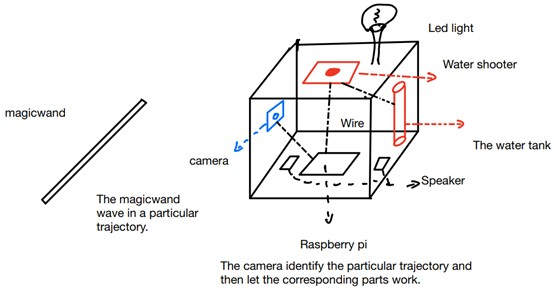As for the whole system, it mainly has four parts and they are Raspberry Pi, the Raspberry camera, the Pi TFT and the LED part. The raspberry is responsable for processing the whonle pragram and issue instructions to various modules of the system. the Raspberry camera is to capture the video stream to the Raspberry pi. In addition, the Led part is to show the magic spell in a more clear way. Pi TFT is used to show the game process.

And for the running process, at first the camera will capture video stream and pass it to Raspberry Pi. Then the Pi change the frames to gray scales and then we call a function called "cv2.HoughCircles" to detect circle (the reason is that the front end of wand is circle). And next it will compare the coordinates of circle in current frame with that in last frame. Finally the result of this comparison will give us an idea of the direction in which the wand is moving and print the corresponding spells. In addition the pygame module will show the game process on PiTFF.

Overall schematic circuit diagram of Magic Potter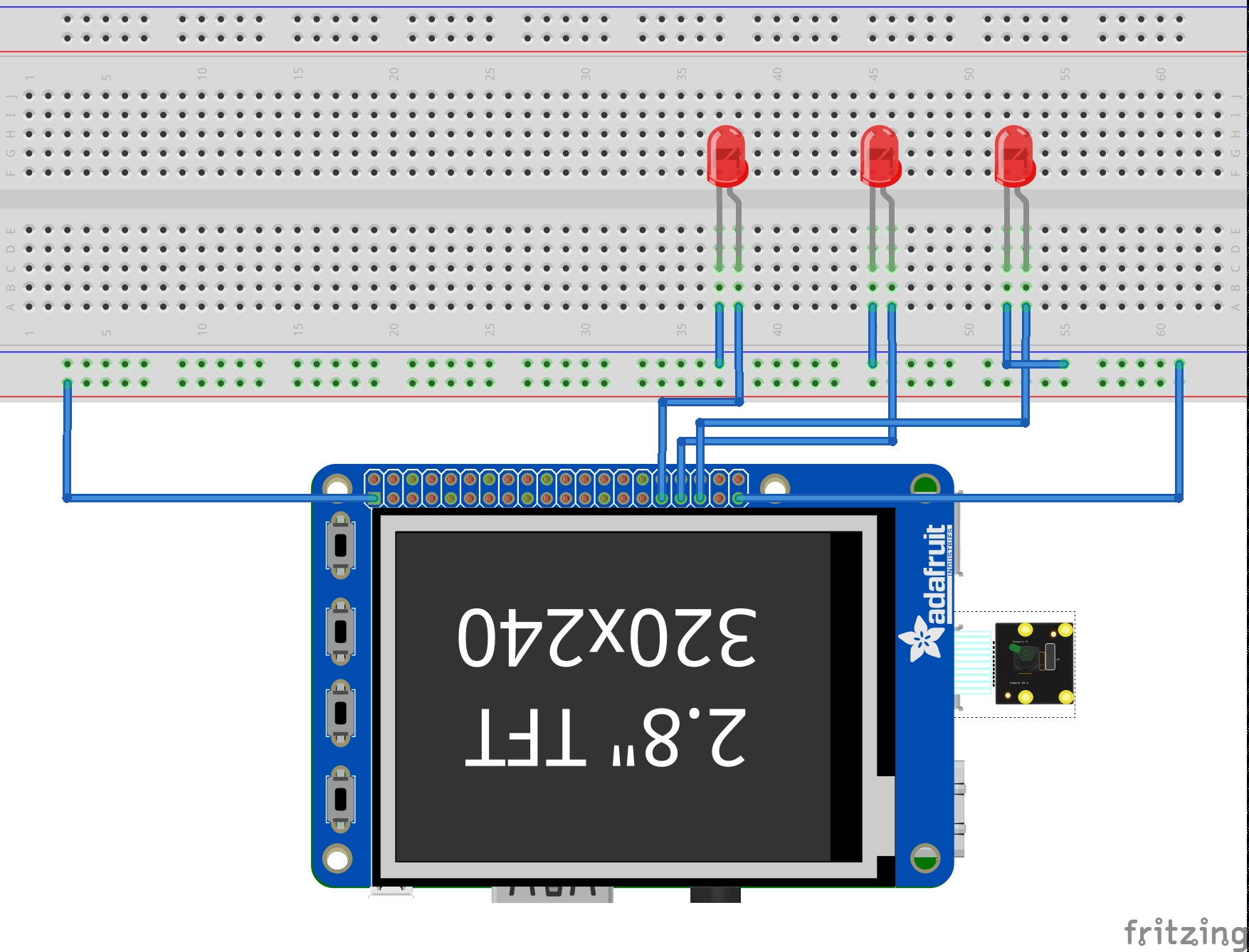## Drawings

As for the overall structure, we use a box to cover the system parts, and the final demo is shown below.

Overall structure of Magic Potter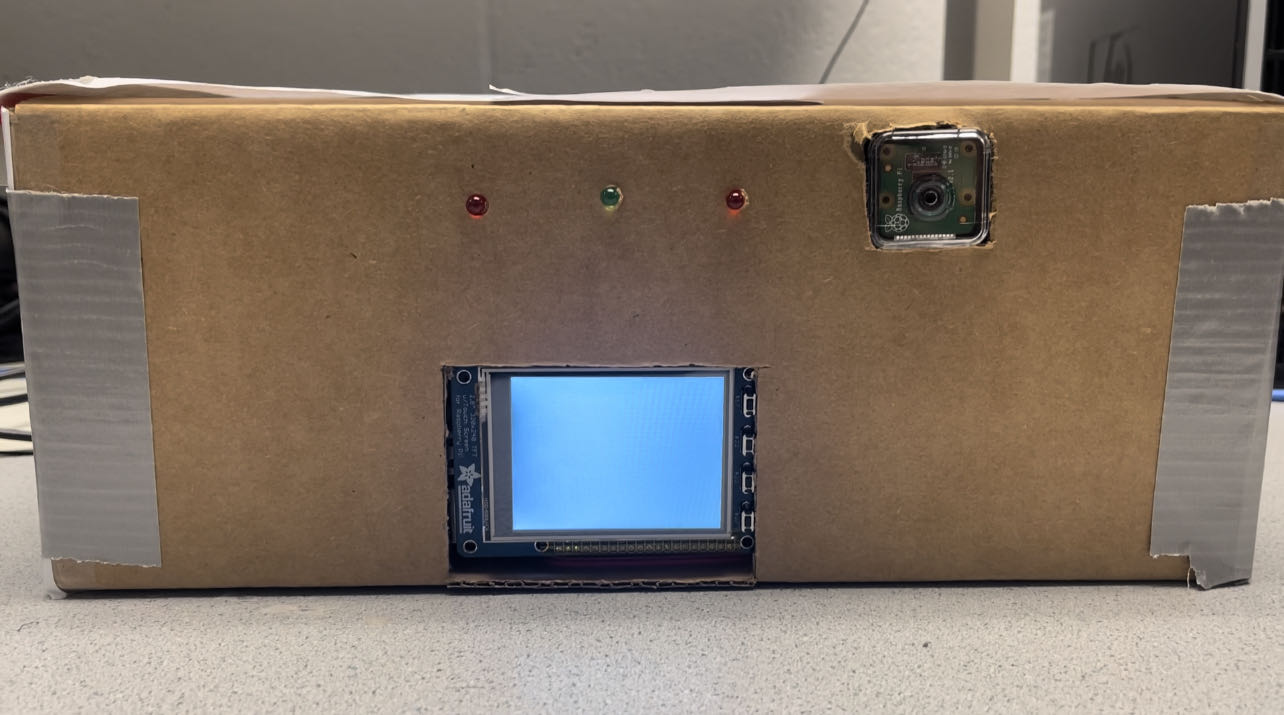Detailed assembling parts

Pi Camera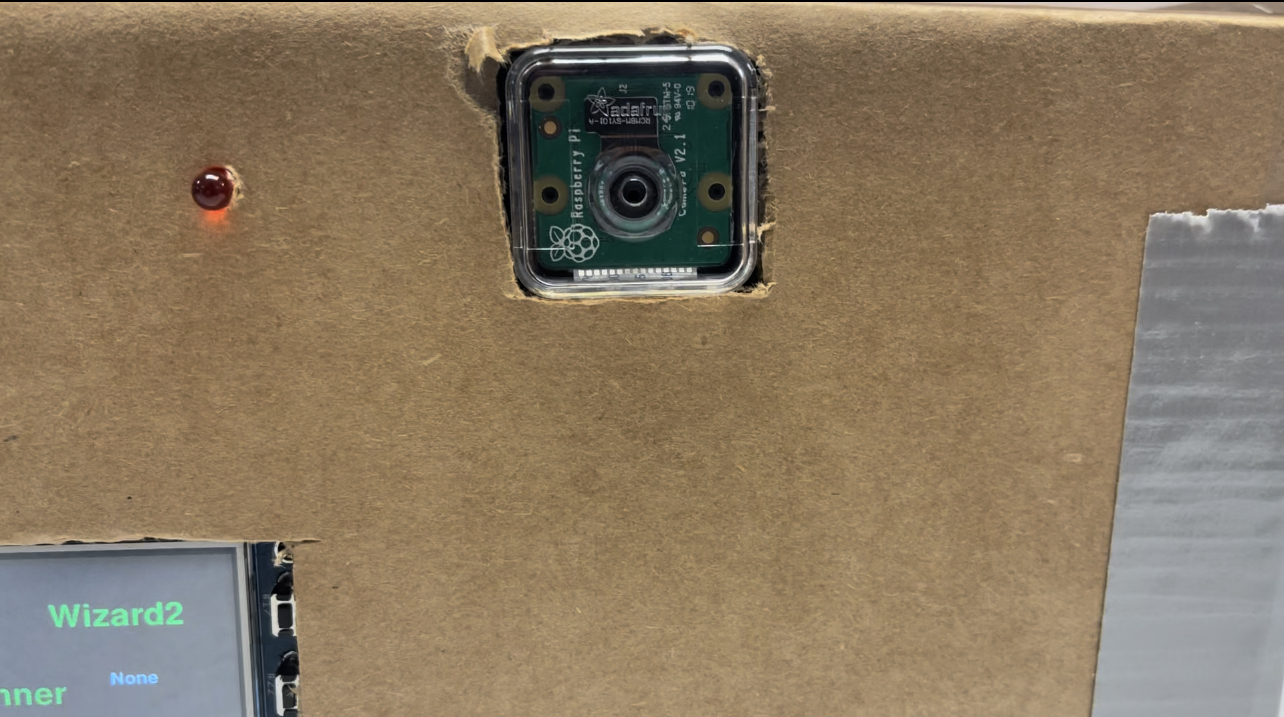PiTFT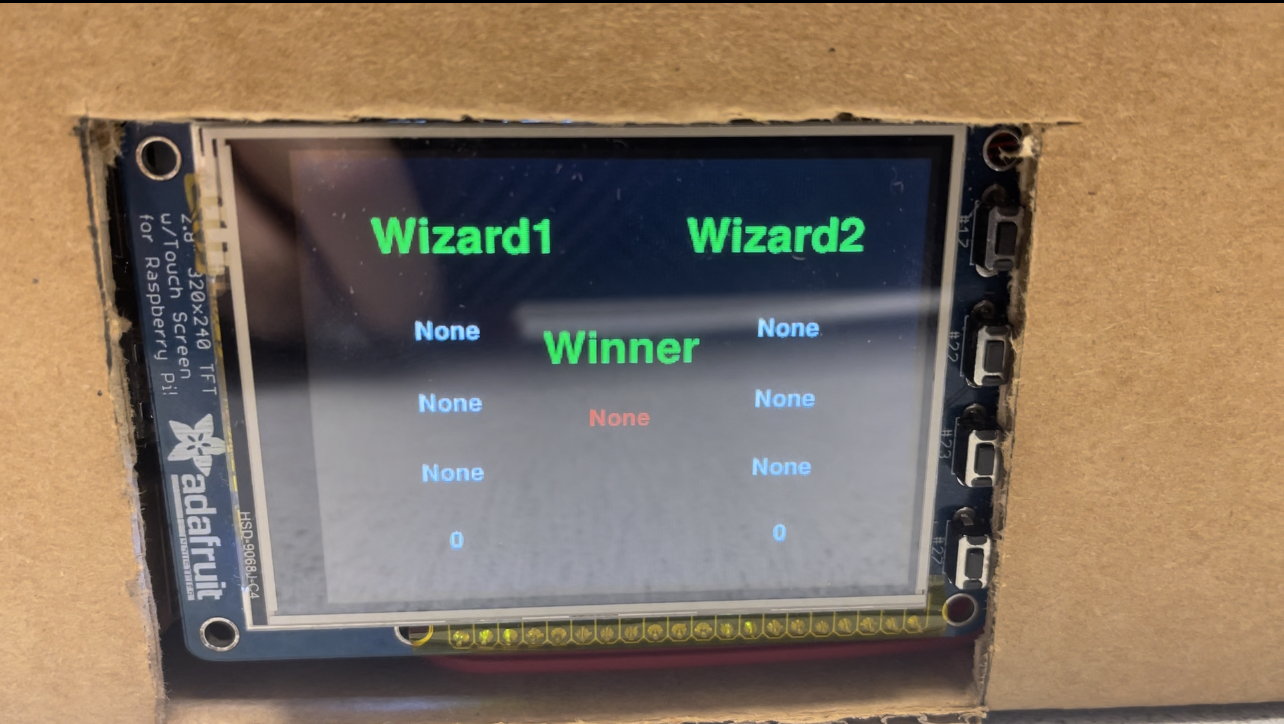LEDs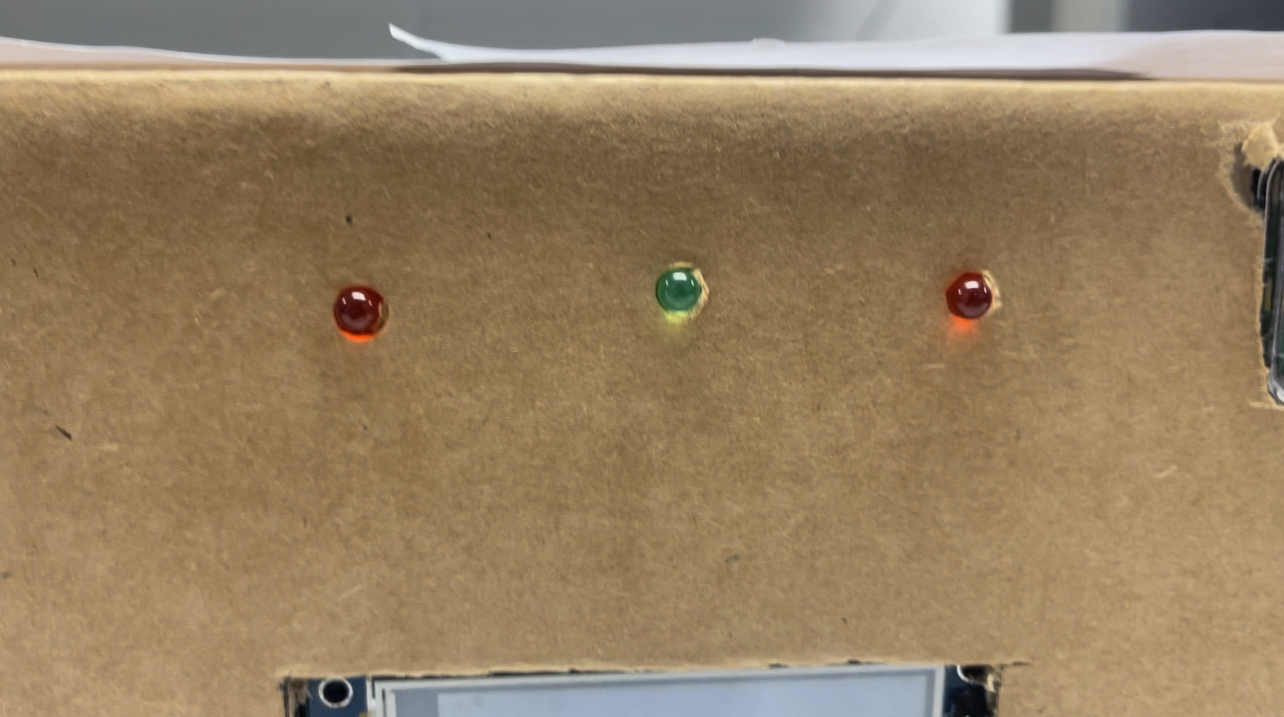## Testing

After we finished the first edition demo. we have several steps to test the functions of the system. First of all, we used different speed to wave the magic wand to test the the recognition accuracy of the camera, then we found at low speed the trajectory could be correctly recognized. In addition, we tested that if the leds could separately light up corresponding to various trajectory. The resuls are satisfactory, which means we can add more action to the system, such as controlling a motor to water a plant. Thirdly we tested the the legibility of the content displayed by PiTFT, and tested if the physical button is working smoothly (we use physical button to restart the game). At last, we ran the whole system to see if the game is running correctly.

## Result

As for the project result, we successfully achieve the functions we had envisioned. The system can implement the recognition of different trajectory of magic wand, and can successfully run the game. When the player move the wand in a slow and stable speed, the spells will be recognized precisely, and the game process shown on Pi TFT are detailed. Overall, the project results are satisfactory, and the deficiencies of the project can be further upgraded and adjusted in the later period.

## Future Work

As a summary, we finally can smoothly run the game with two players. And the camera can successfully recognize the trajectory of wand in most cases. However, the recognition accuracy of the camera is still not enough, which we believe is that the performance of the currently used algorithm cannot meet our expected requirements. After exploration, we find if we capture more feature points in each frame and compare the current frame with the last one. And then calculate the coordinates' movement of similar feature points to obtain the direction of wand. In this method, it can get better results than that of current algorithm. So that in the future, we plan to update the algorithm, use a better camera and some brighter leds. All in all, we achieve the preconceived goals.

## Work Distribution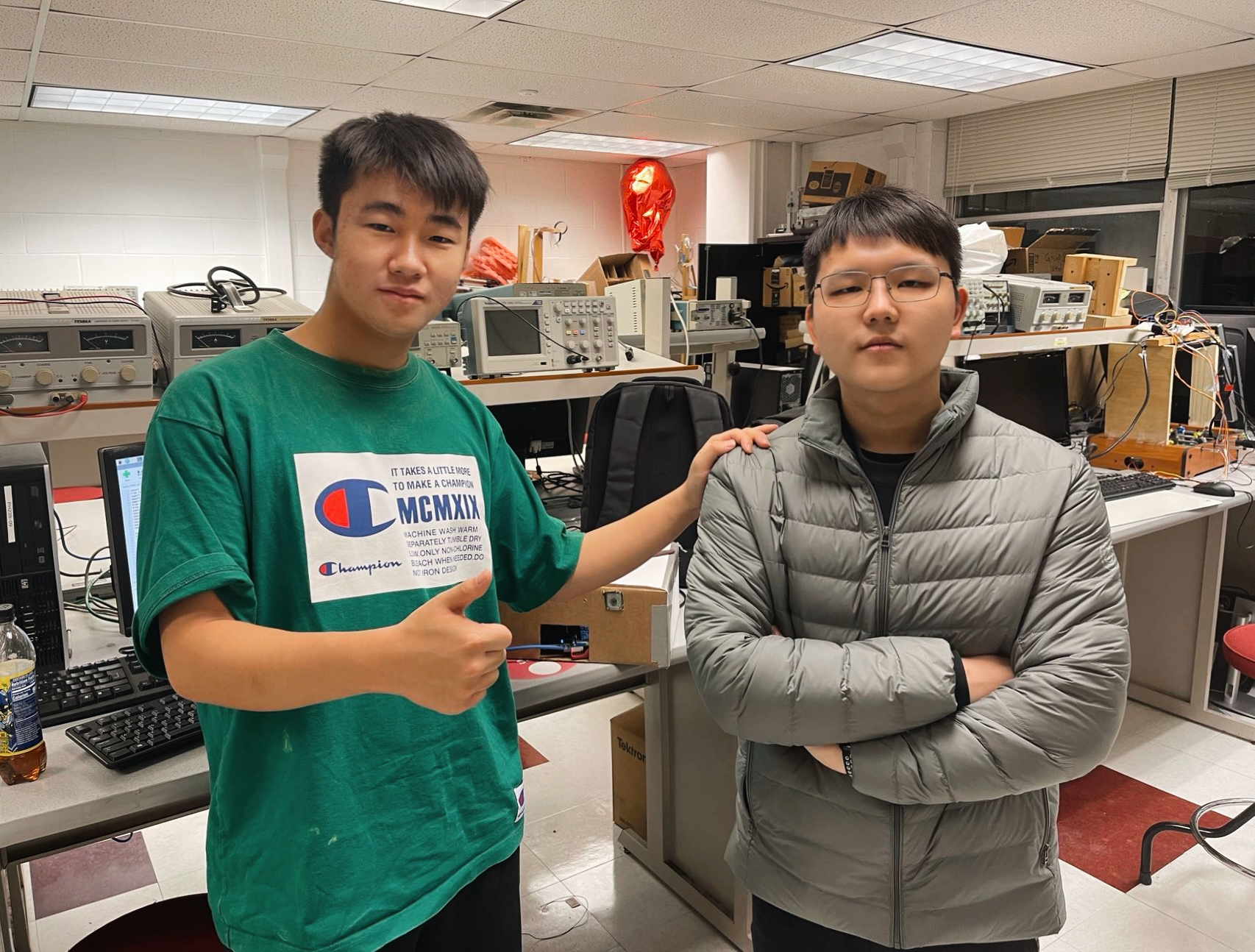#### Project group picture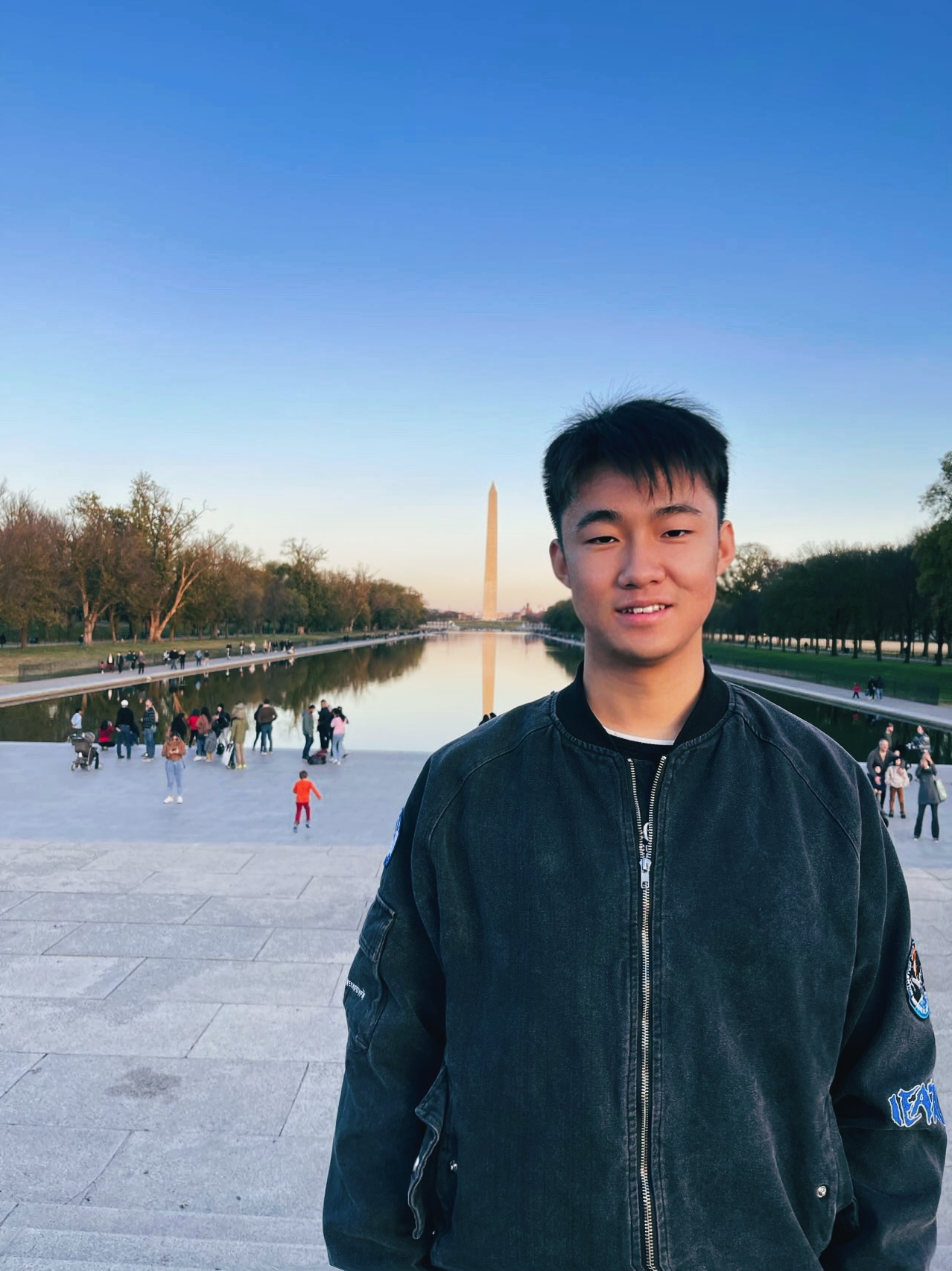### Huan Yang(hy468)

hy468@cornell.edu

Designed parts of the software architecture. Tested the whole system. Made parts of website, project report and demonstration video.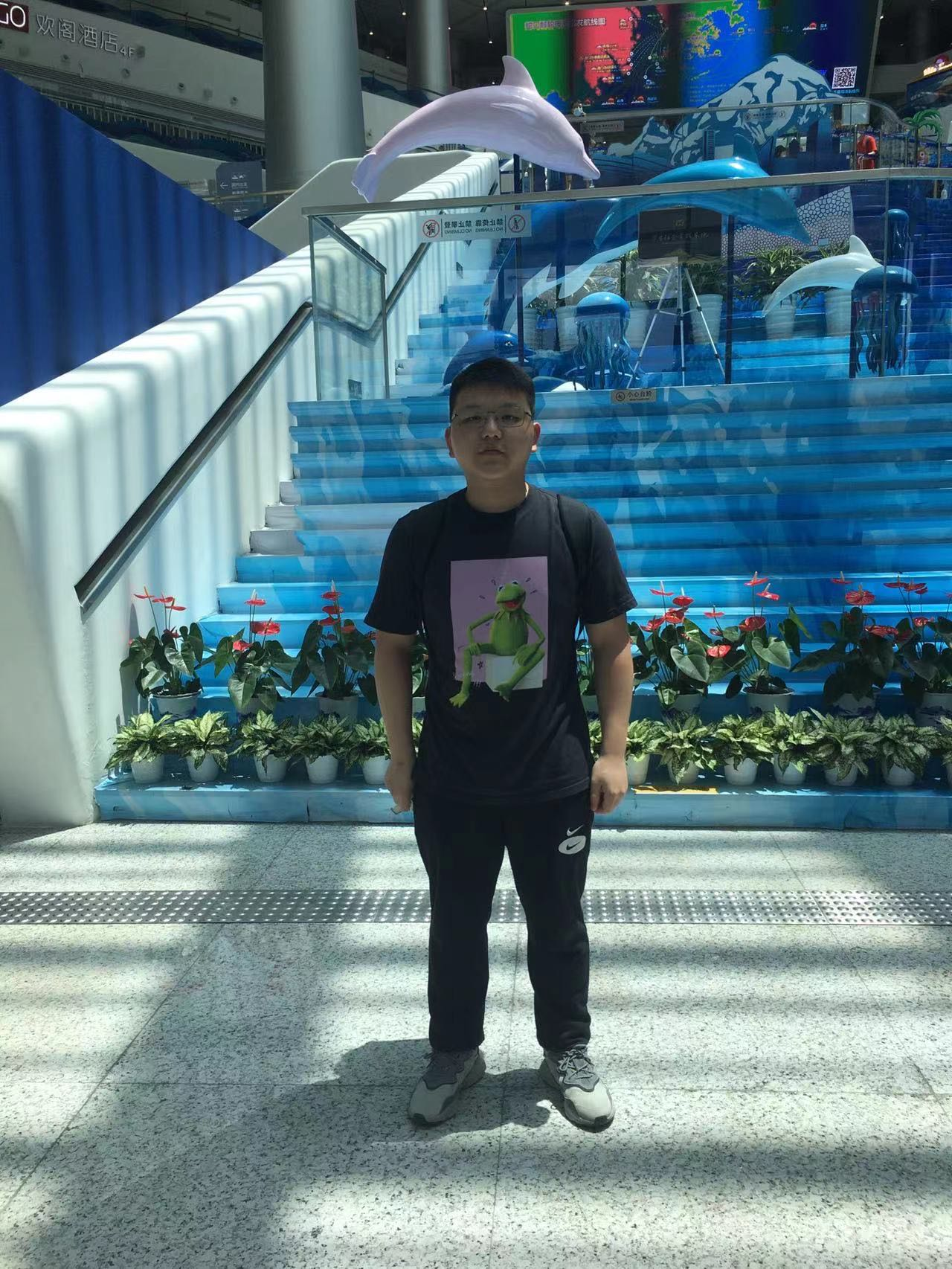### Hao Chen(hc798)

hc795@cornell.edu

Designed parts of software architecture. Tested the whole system. Made parts of website, project report and demonstration video.

## Parts List

• Raspberry Pi \$35.00
• Raspberry Pi Camera V2 \$25.00
• LEDs, boxes, Resistors and Wires - Provided in lab

## References

PiCamera Document
OpenCV
Pygame
Pigpio Library
R-Pi GPIO Document

## Code Appendix

```    import io
from io import BytesIO
import sys
import pygame
import os
from pygame.locals import *
from picamera import PiCamera
import numpy as np
import cv2
import math
import time
import pigpio
import RPi.GPIO as GPIO

GPIO.setmode(GPIO.BCM)
GPIO.setup(5, GPIO.OUT)
GPIO.setmode(GPIO.BCM)
GPIO.setup(13, GPIO.OUT)
GPIO.setmode(GPIO.BCM)
GPIO.setup(19, GPIO.OUT)
GPIO.setup(26, GPIO.OUT)
GPIO.setup(23, GPIO.IN, pull_up_down=GPIO.PUD_UP)
GPIO.setup(27, GPIO.IN, pull_up_down=GPIO.PUD_UP)

os.putenv('SDL_VIDEODRIVER', 'fbcon')
os.putenv('SDL_FBDEV', '/dev/fb0')
os.putenv('SDL_MOUSEDRV', 'TSLIB')
os.putenv('SDL_MOUSEDEV', '/dev/input/touchscreen')
pygame.init()
pygame.mouse.set_visible(False)

WHITE = 255, 255, 255
RED = 255, 0, 0
GREEN = 0, 255, 0
BLACK = 0, 0, 0

my_buttons = {'Wizard1':(80,40), 'Wizard2':(240,40), 'Winner':(160,100)}
winner = {(160,140):'None'}
left_buttons = {(70,90):'None', (70, 130):'None', (70,170):'None', (70,210):'0'}
right_buttons = {(250,90):'None', (250, 130):'None', (250,170):'None', (250,210):'0'}
my_buttons_rect = {}
my_font = pygame.font.Font(None, 35)
my_font1 = pygame.font.Font(None, 20)
my_font2 = pygame.font.Font(None, 30)
screen = pygame.display.set_mode((320,240))
screen.fill(BLACK)
flag = True
flag1 = False
flag2 = False

def GPIO5():#the defination of GPIO
start = time.time()
end = time.time() + 3
while end - start > 0:
GPIO.output(5, GPIO.HIGH)
time.sleep(0.1)
GPIO.output(5, GPIO.LOW)
time.sleep(0.1)
start = time.time()

GPIO.output(5, GPIO.LOW)

def GPIO13():
start = time.time()
end = time.time() + 3
while end - start > 0:
GPIO.output(13, GPIO.HIGH)
time.sleep(0.1)
GPIO.output(13, GPIO.LOW)
time.sleep(0.1)
start = time.time()

GPIO.output(13, GPIO.LOW)

def GPIO19():
start = time.time()
end = time.time() + 3
while end - start > 0:
GPIO.output(19, GPIO.HIGH)
time.sleep(0.1)
GPIO.output(19, GPIO.LOW)
time.sleep(0.1)
start = time.time()

GPIO.output(19, GPIO.LOW)

def GPIO26():
GPIO.output(26, GPIO.HIGH)

def Scan():#call Scan will run find wand
global flag
while flag:
FindNewPoints()

def FindNewPoints():#find the circle similar to the front end of wand shown in the video stream
ig = [ for x in range(20)]
TrackWand()

def TrackWand():
color = (0,0,255)
cam.capture(stream, 'jpeg')#capture a photo file to check the camera view
data = np.fromstring(stream.getvalue(), dtype=np.uint8)
old_frame = cv2.imdecode(data, 1)
cv2.flip(old_frame,1,old_frame)
old_gray = cv2.cvtColor(old_frame, cv2.COLOR_BGR2GRAY)

# find the first frame and find circles in it

p0.shape = (p0.shape, 1, p0.shape)
p0 = p0[:,:,0:2]

while flag:
my_stream = BytesIO()
cam.capture(my_stream, 'jpeg')
data2 = np.fromstring(my_stream.getvalue(), dtype=np.uint8)
frame = cv2.imdecode(data2, 1)
cv2.flip(frame,1,frame)
frame_gray = cv2.cvtColor(frame, cv2.COLOR_BGR2GRAY)

p1, st, err = cv2.calcOpticalFlowPyrLK(old_gray, frame_gray, p0, None, **lk_params)#calculate the video optical flow
# Select good points
good_new = p1[st==1]
good_old = p0[st==1]
# draw the tracks
for i,(new,old) in enumerate(zip(good_new,good_old)):
a,b = new.ravel()  # a,c-the x-axis of the center， b,d-the y-axis of the center
c,d = old.ravel()

if (i<10):# the maximum center of circle can be detected is 10
IsGesture(a,b,c,d,i)

if flag1:
break

flag1 = False#set flags to run the while
cam.capture(my_stream, 'jpeg')
data3 = np.fromstring(my_stream.getvalue(), dtype=np.uint8)
frame = cv2.imdecode(data3, 1)
#update the frames and points
old_gray = frame_gray.copy()#copy the gray frame
p0 = good_new.reshape(-1,1,2)

def Spell(spell):
global num,flag,lupdate,rupdate

ig = [ for x in range(8)]
if (spell=="Colovaria"):
if (num % 2) == 1:
lupdate = spell
left()
elif (num % 2) == 0:
rupdate = spell
right()
num += 1
flag = False
print('flag is False')
print('Colovaria','leftdown')
print(astr)
GPIO5()

elif (spell=="Lumos"):
if (num % 2) == 1:
lupdate = spell
left()
elif (num % 2) == 0:
rupdate = spell
right()
num += 1
flag = False
print('flag is False')
print('Lumos','rightdown')
print(astr)
GPIO13()

elif (spell=="Nox"):
if (num % 2) == 1:
lupdate = spell
left()
elif (num % 2) == 0:
rupdate = spell
right()
num += 1
flag = False
print('flag is False')
print('Nox','rightup')
print(astr)
GPIO19()

Scan()

def IsGesture(a,b,c,d,i):
global flag1, astr
print("point: %s" % i)# add the movement we recognized into a list

if ((a<(c-4)) and (abs(b-d)<2)):
ig[i].append("left")
elif ((c<(a-4)) and (abs(b-d)<2)):
ig[i].append("right")
elif (b<(d-6)):
ig[i].append("up")
elif (d<(b-6)):
ig[i].append("down")

astr = ''.join(map(str, ig[i]))
if "rightdown" in astr:
Spell("Lumos")
flag1 = True
elif "rightup" in astr:
Spell("Nox")
flag1 = True
elif "leftdown" in astr:
Spell("Colovaria")
flag1 = True
print(astr)

def left():
left_buttons[(70,90)] = left_buttons[(70,130)]
left_buttons[(70,130)] = left_buttons[(70,170)]
left_buttons[(70,170)] = lupdate

def right():
right_buttons[(250,90)] = right_buttons[(250,130)]
right_buttons[(250,130)] = right_buttons[(250,170)]
right_buttons[(250,170)] = rupdate

def compare():
lvalue = left_buttons[(70,170)]
rvalue = right_buttons[(250,170)]

if lvalue == 'Nox' and rvalue == 'Lumos':
left_buttons[(70,210)] = str(int(left_buttons[(70,210)]) + 1)
elif lvalue == 'Lumos' and rvalue == 'Nox':
right_buttons[(250,210)] = str(int(right_buttons[(250,210)]) + 1)

elif lvalue == 'Lumos' and rvalue == 'Colovaria':
left_buttons[(70,210)] = str(int(left_buttons[(70,210)]) + 1)
elif lvalue == 'Colovaria' and rvalue == 'Lumos':
right_buttons[(250,210)] = str(int(right_buttons[(250,210)]) + 1)

elif lvalue == 'Colovaria' and rvalue == 'Nox':
left_buttons[(70,210)] = str(int(left_buttons[(70,210)]) + 1)
elif lvalue == 'Nox' and rvalue == 'Colovaria':
right_buttons[(250,210)] = str(int(right_buttons[(250,210)]) + 1)

def getwinner():
lvalue = int(left_buttons[(70,210)])
rvalue = int(right_buttons[(250,210)])

if lvalue == rvalue:
winner[(160,140)] = 'doffall'

elif lvalue > rvalue:
winner[(160,140)] = 'Wizard1'

elif lvalue < rvalue:
winner[(160,140)] = 'Wizard2'

def restart():
global winner, left_buttons, right_buttons, num
winner = {(160,140):'None'}
left_buttons = {(70,90):'None', (70, 130):'None', (70,170):'None', (70,210):'0'}
right_buttons = {(250,90):'None', (250, 130):'None', (250,170):'None', (250,210):'0'}
num = 1

lk_params = dict( winSize  = (15,15),
maxLevel = 2,                                                          # cv2.TERM_CRITERIA_COUNT iterate 10 times
criteria = (cv2.TERM_CRITERIA_EPS | cv2.TERM_CRITERIA_COUNT, 10, 0.03)) # cv2.TERM_CRITERIA_EPS
dilation_params = (5, 5)
movment_threshold = 80
num = 1
lupdate = ''
rupdate = ''
stream = BytesIO()   # Create an in-memory stream
cam = PiCamera()    # initialize
cam.framerate = 24    # set frequency

def End():
cam.close()

while True:
flag = True

screen.fill(BLACK)

if num == 3 or num == 5 or num == 7:
compare()
if num == 7:
num += 1

if num == 8:
getwinner()
flag2 = True

for my_text, text_pos in my_buttons.items():
text_surface = my_font.render(my_text, True, GREEN)
rect = text_surface.get_rect(center=text_pos)
screen.blit(text_surface, rect)

for text_pos, my_text in left_buttons.items():
text_surface = my_font1.render(my_text, True, WHITE)
rect = text_surface.get_rect(center=text_pos)
screen.blit(text_surface, rect)

for text_pos, my_text in right_buttons.items():
text_surface = my_font1.render(my_text, True, WHITE)
rect = text_surface.get_rect(center=text_pos)
screen.blit(text_surface, rect)

for text_pos, my_text in winner.items():
text_surface = my_font1.render(my_text, True, RED)
rect = text_surface.get_rect(center=text_pos)
screen.blit(text_surface, rect)

if (not GPIO.input(27)):
sys.exit()

pygame.display.flip()

if num <= 6:
Scan()

while flag2:
if (not GPIO.input(27)):
sys.exit()

if (not GPIO.input(23)):
restart()
flag2 = False

```